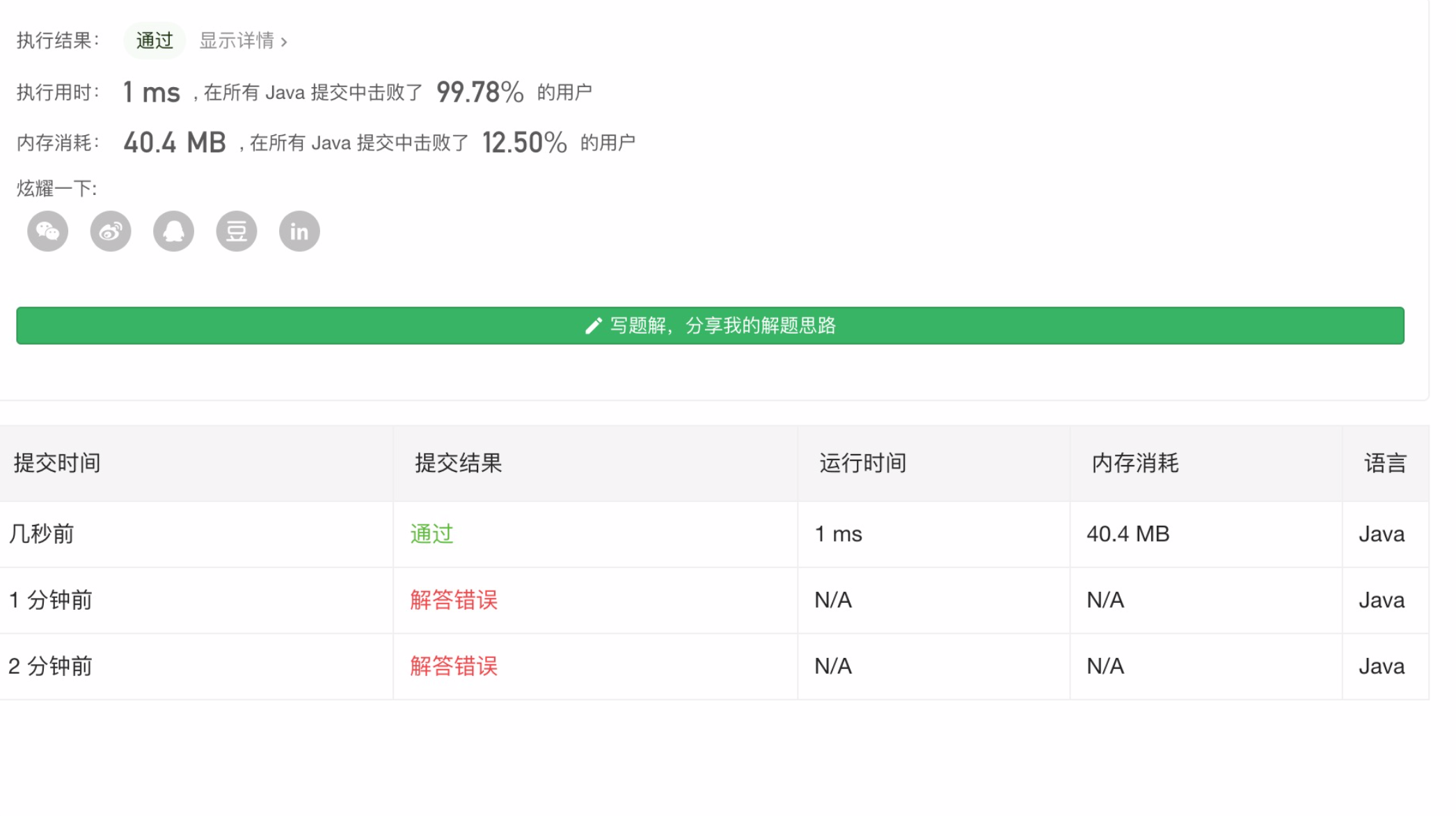### 题干：

示例 1:

示例 2:

### 代码：

 // 674
public static int findLengthOfLCIS(int[] nums) {
if (nums.length == 0 || nums == null) {
return 0;
}
int lastCount = 1;
int count = 1;
for (int i = 0; i < nums.length - 1; i++) {
if (nums[i] < nums[i + 1]) {
count++;
} else {
if (count > lastCount) {
lastCount = count;
}
count = 1;
}
}
return Math.max(lastCount, count);
}Q.E.D.